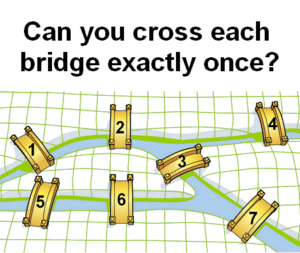Home -> Solved problems -> Is the walk possible?

## Is the walk possible?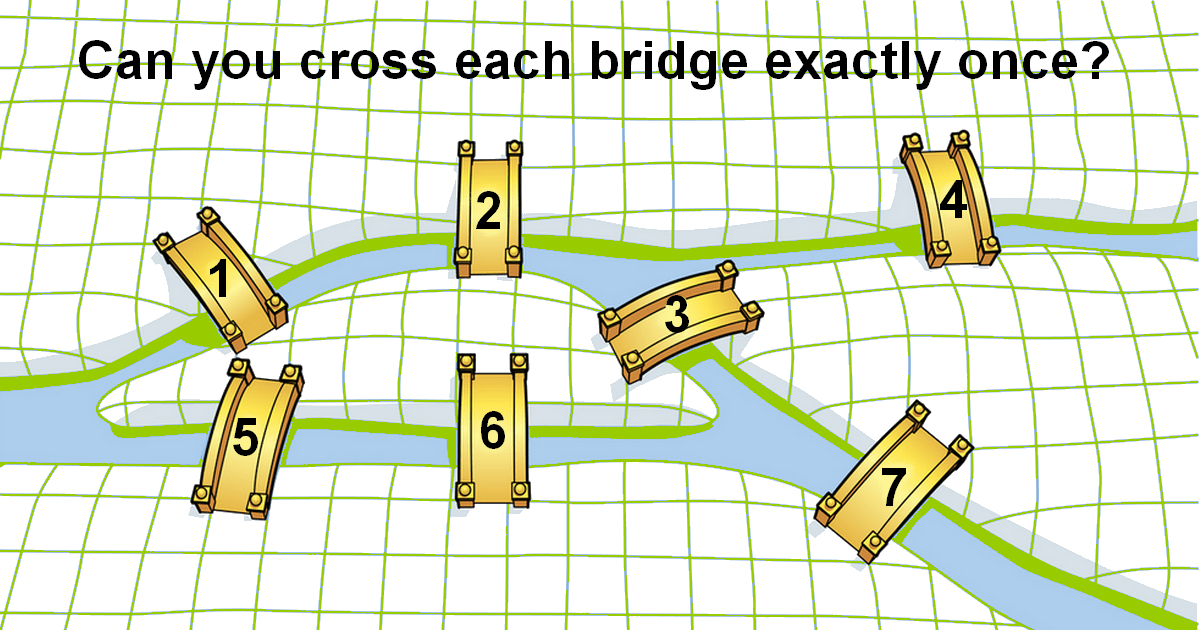### Solution

The solution is proposed by the Swiss mathematician Leonhard Euler. Let’s start

To solve the problem Leonhard Euler started with the assumption that the walk is possible. For one confrontation with a particular landmass, different than the initial or final one, two different bridges must be taken into consideration, one bridge for entry to the landmass and the other one for exiting it. Therefore, each landmass must be an endpoint of an amount of bridges equaling two times the number of how many times it is confronted over the walk. Consequently, each landmass, with the possibility of exception of the initial and final ones if they are different, must be an endpoint of an even amount of bridges. Now, for our example which is known as Bridges of Königsberg we have one landmass as an endpoint of five bridges and the others are endpoints of three bridges. Therefore, the walk is not possible.
Home -> Solved problems -> Is the walk possible?

### Related Topics

Find the volume of the square pyramid as a function of $$a$$ and $$H$$ by slicing method.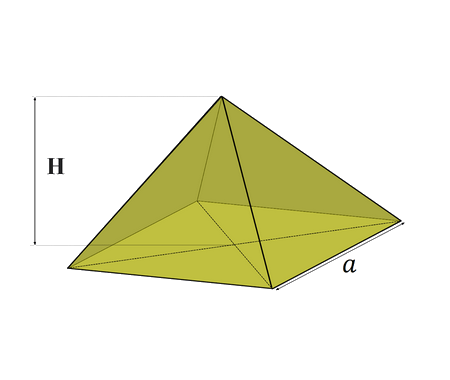Prove that $\lim_{x \rightarrow 0}\frac{\sin x}{x}=1$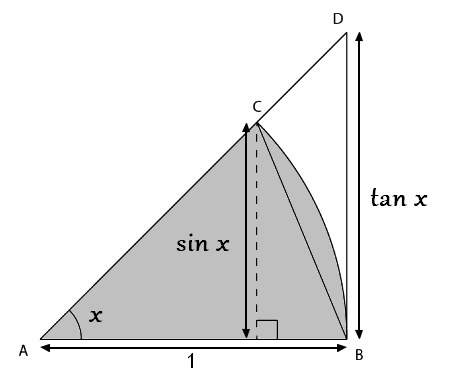Prove that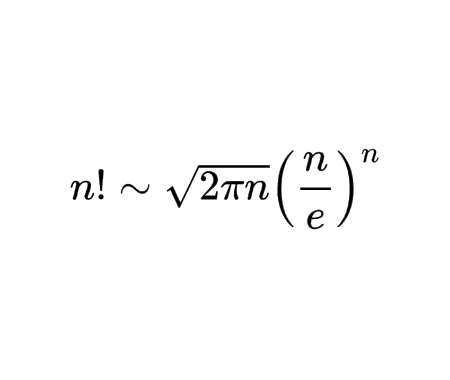Calculate the half derivative of $$x$$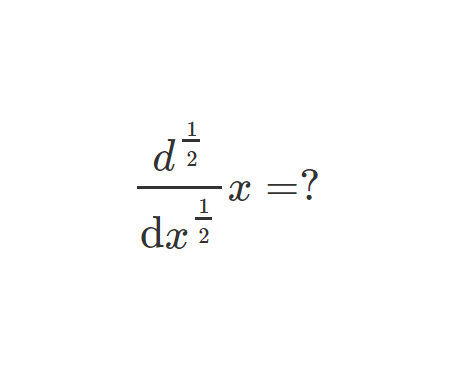Prove Wallis Product Using Integration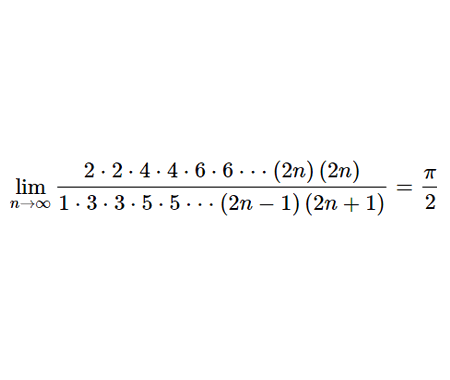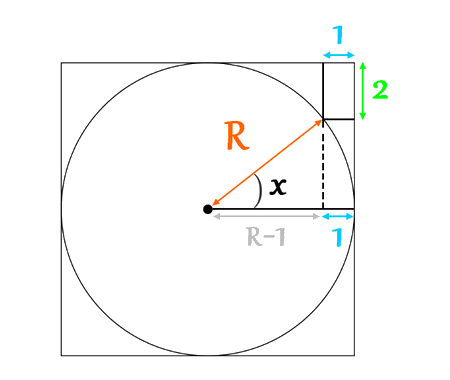Calculate the volume of Torus using cylindrical shells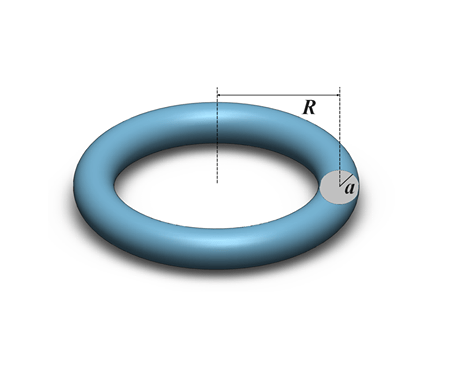Find the derivative of exponential $$x$$ from first principles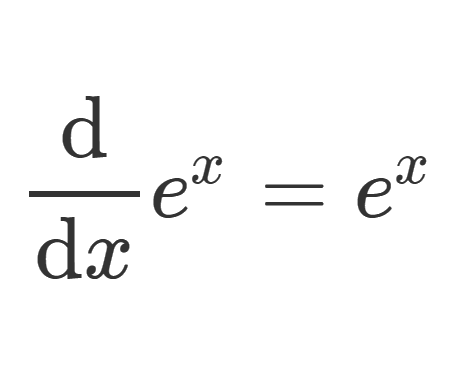Calculate the sum of areas of the three squares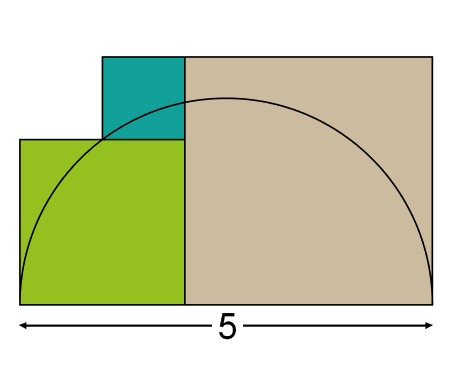Find the equation of the curve formed by a cable suspended between two points at the same height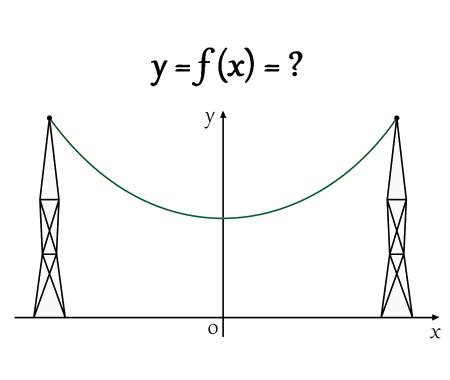Solve the equation for real values of $$x$$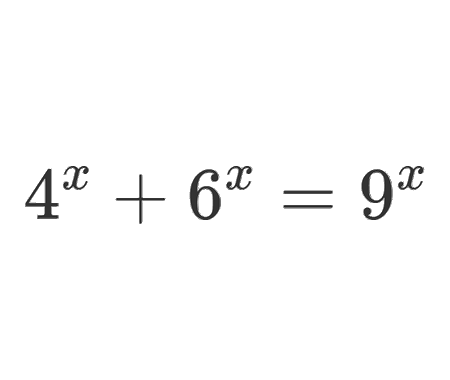Solve the equation for $$x\epsilon\mathbb{R}$$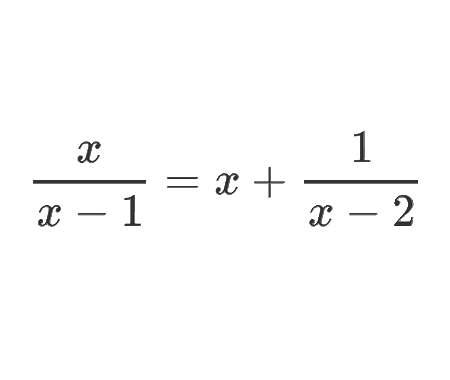Determine the angle $$x$$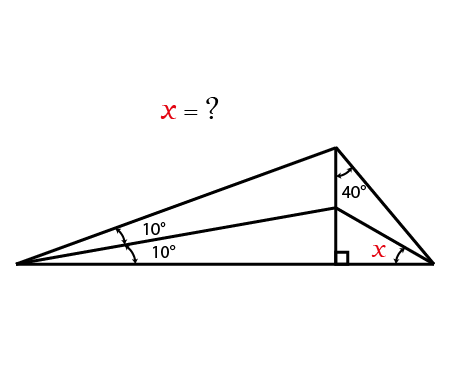Calculate the following limit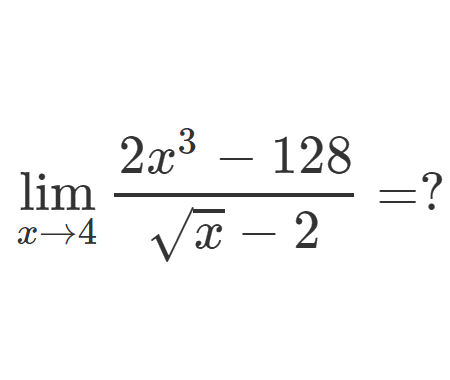Calculate the following limit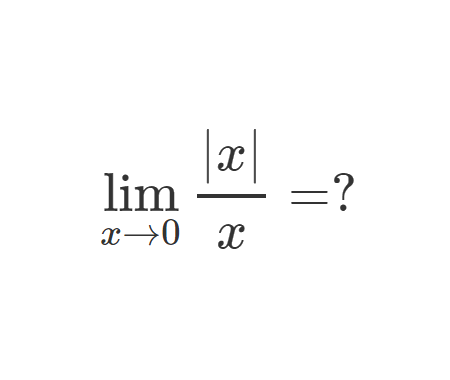Calculate the integral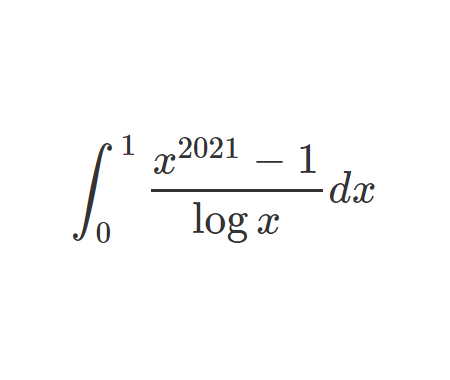Challenging problem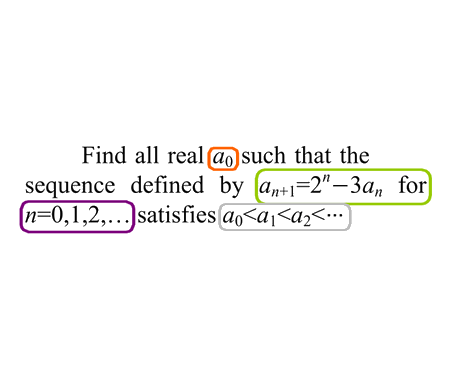Prove that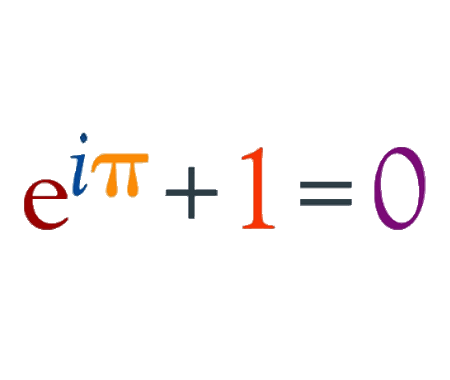Prove that $$e$$ is an irrational numberFind the derivative of $$y$$ with respect to $$x$$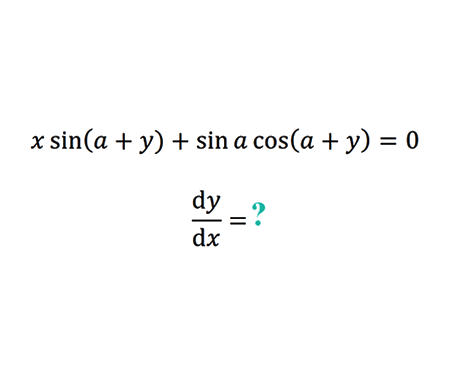Find the limit of width and height ratio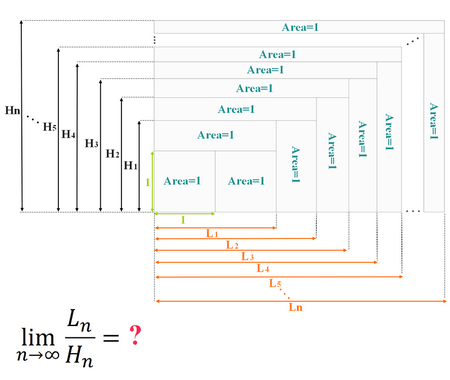How Tall Is The Table ?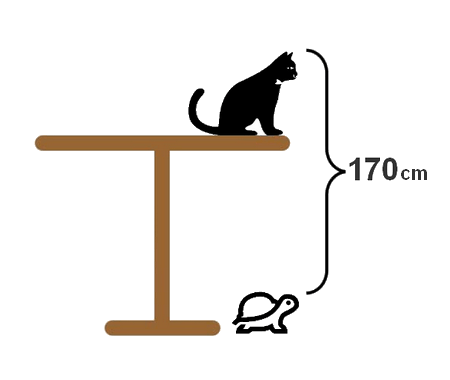Why 0.9999999...=1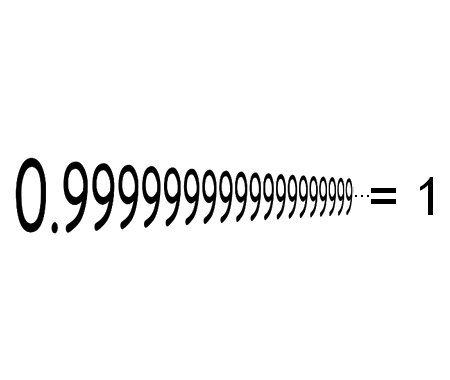Solve the equation for $$x \in \mathbb{R}$$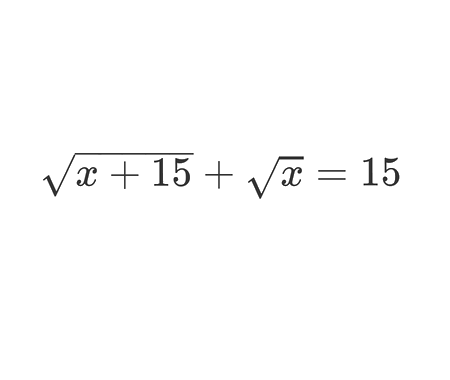Is the walk possible?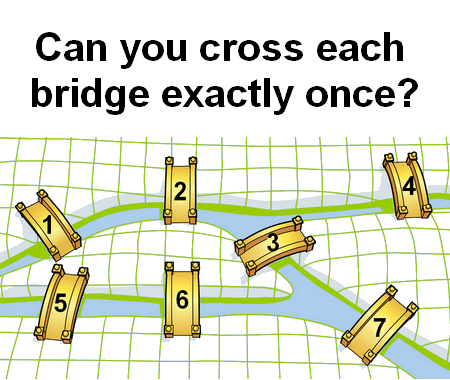Home -> Solved problems -> Is the walk possible?

#### Share the solution: Is the walk possible?